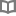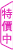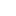急不來時，你可以按一下暫停鍵 - 朱成玉 - BOOKWALKER中文電子書

急不來時，你可以按一下暫停鍵

作者： 朱成玉

•朱成玉

百花散文獎得主。在文筆中展現了日益圓融、成熟的寫作功力。寫故事娓娓道來，寫人物栩栩如生，寫情感濃淡相間，寫道理自然深刻，讀起來輕鬆而又有強烈的獲得感。迄今已發表各類作品500餘萬字。已出版作品《一生只有七天》、《給痛苦一個流淌的出口》、《向美好的舊日時光道歉》等三十餘部。

• 放入購物車
•試閱

• 放入購物車
•試閱

• 放入購物車
•試閱

• 放入購物車
•試閱• 放入購物車
•試閱

• 放入購物車
•試閱

• 放入購物車
•試閱

• 放入購物車
•試閱• 放入購物車
•試閱

• 放入購物車
•試閱

• 放入購物車
•試閱

• 放入購物車
•試閱• 放入購物車
•試閱

• 放入購物車
•試閱

• 放入購物車
•試閱

• 放入購物車
•試閱

• 放入購物車
•試閱

• 放入購物車
•無試閱

• 放入購物車
•試閱

• 放入購物車
•試閱

• 放入購物車
•試閱

• 放入購物車
•試閱

• 放入購物車
•試閱

• 放入購物車
•試閱

• 放入購物車
•試閱

• 放入購物車
•試閱

• 放入購物車
•試閱• 放入購物車
•試閱

• 放入購物車
•試閱

• 放入購物車
•試閱

• 放入購物車
•試閱

• 放入購物車
•試閱

• 放入購物車
•試閱

• 放入購物車
•試閱

• 放入購物車
•試閱

• 放入購物車
•試閱

• 放入購物車
•試閱

• 放入購物車
•試閱

• 放入購物車
•試閱

• 放入購物車
•試閱• 放入購物車
•試閱• 放入購物車
•試閱

• 放入購物車
•試閱

• 放入購物車
•試閱